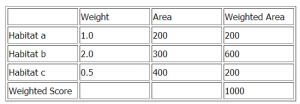# Value Models

A weighted index or a value model is a scale that weights and combines different impacts. For example, if there are different types of affected wildlife habitat, calculate a weighted summary score or index of the overall effect by:

Estimating the impact on each criterion (each habitat type) under each alternative.

Assigning a weight (or importance) to the impact (W).

Calculating a score for each alternative that is the weighted sum across habitat types (a, b, c):

Score for Alternative 1 = Wa x Aa + Wb x Ab + Wc x Ac

(click image to enlarge)In general, the steps for developing a weighted index are:

Estimate the impact on each criterion for each alternative. This is a technical judgment.

Assign weights to each criterion. Assign them only once. This is a mix of value and technical judgments.

Use the weighted score (1000) to compare alternatives. It can be interpreted as weighted hectares, or in this case, equivalent hectares of Habitat a.

If the attributes are reported in different units, then you must normalize criterion scores before weighting.

### Key Ideas

Value models help summarize multiple impacts into a single index